# Relations

Society does not consist of individuals, but expresses the sum of interrelations, the relations within which these individuals stand.

Karl Marx, Grundrisse der Kritik der Politischen Ökonomie

If two young people are seeing each other romantically, we usually say something like Frank and Jeremy are in a relationship. The word in suggests we should use the notion of set membership to record what a relationship means.

### Overview of Relations

• Relations and Set Products (this page) Relations are sets of pairs, so we must think about sets of pairs.
• Basics of Relations.
• Operations on Relations. Relations can be combined in interesting ways.
• Graphing Relations. We can draw visual representations of relations in several different ways.
• Properties of Relations.
• Equivalence Relations. Relations which generalize.
• Ordering Relations. Relations which generalize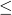.

### Pairs of Elements, Products of Sets

Since a relation is a set of pairs, let's try to understand sets of pairs.

Definition. The ordered pair with coordinates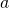and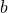is the symbol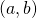. Two ordered pairsand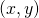are the same iff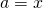and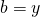.

Definition. The product of sets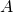and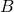is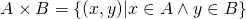That is,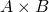is the set of all pairs whose first coordinate comes fromand whose second coordinate comes from.

We've seen set products before: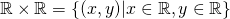is the set of all pairs of real numbers, i.e. the Cartesian plane. In factis sometimes called the Cartesian product ofand. (Both the plane and the more general product are named for the philosopher and mathematician Rene Descartes.)

Sinceis a set, we should ask, what does it mean to be an element of? That is, let's unpack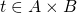. This should mean:But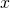and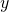are new variables, so they require new quantifiers. In fact,means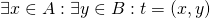Theorem. For any sets,,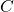, and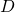,

•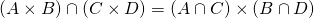•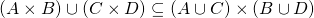Note that the second clause is not set equality, merely subset. This is not an omission.

Proof. We'll prove the first clause and leave the second as an exercise.

First we'll show that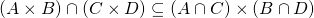. To this end, let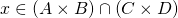. So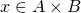and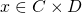. Then there are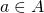and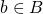with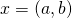. On the other hand, there are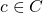and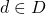with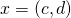. Since, we see that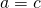and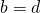. So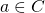, hence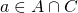. Similarly,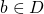, so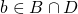. Thus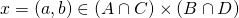.

Now we'll show the other inclusion,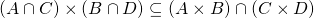. To this end, let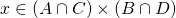. Then there are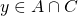and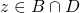with. Since, we knowand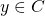. Similarly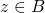and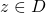. So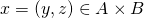and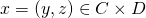, hence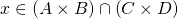.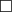Exercise. Is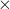commutative or associative? That is, is either of the following equalities automatic?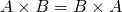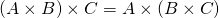There's one more thing to note about set products, which is why we use the word product at all:

Theorem. Ifhas exactlyelements andhas exactly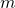elements, thenhas exactly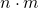elements.

That is, the size of the product is the product of the sizes.